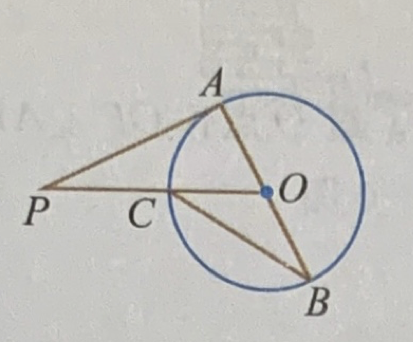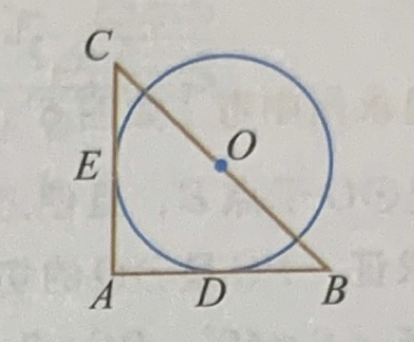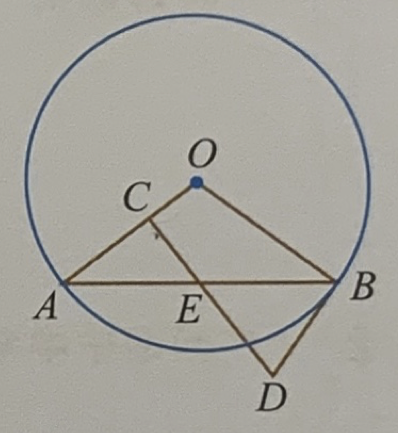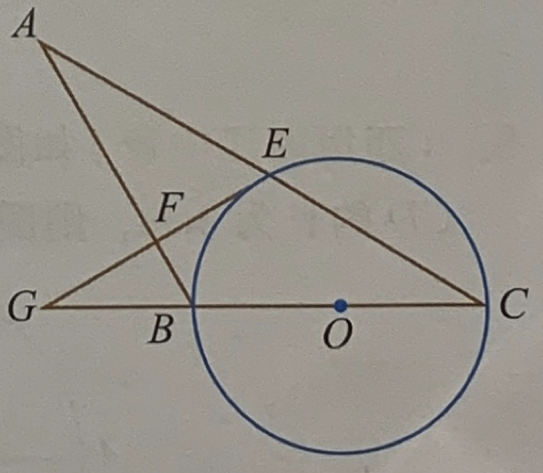# 1 Circle

## 1.1 Basics

1. AB is a diameter of circle $$O$$, $$PA$$ is a tangent line of the circle. $$C$$ is ther intersect point of $$PO$$ and the circle. $$\angle P=40^o$$. What’s $$\angle B$$Figure 1.1: Circle 1

1. The two legs of a right $$\triangle ABC$$ is tagent with a circle $$O$$ whose center $$O$$ is the mid point of the hypotenuse of $$\triangle ABC$$. D and E are the tagent points on $$AB$$ and $$AC$$. $$BC = 2\sqrt 2$$. What’s the length of arc $${DE}$$?Figure 1.2: Circle 2

1. A circle $$O$$ has radius 10, AB and CD are two chords of the circle. $$AB \parallel BC$$, $$AB = 12$$, $$CD=16$$, what’s the distance between AB and CD?

2. Triangle $$ABC$$, $$AB=AC$$, $$\angle ABC = 30^o$$. $$BD$$ is a diameter of $$\triangle ABC$$’s circumsribe circle, $$CD=\frac{4\sqrt 3}{3}$$, AD=?

3. $$AB$$ is a chord of circle $$O$$. $$E$$ is the midpoint of $$AB$$. $$EC\perp OA$$, BD is a tangent line of Circle $$O$$. $$AB=12$$, $$BD=5$$. What is the radius of the circle?Figure 1.3: Circle 5

1. BC is the diameter of circle O with radius 3. Circle O interset with AC at point E. EF is a tangent line of Circle O and $$EF \perp AB$$ at point F. EF is extended and intersect with CB’s extended line at G,$$\angle ABG=2\angle C$$, $$\sin \angle EGC = \frac{3}{5}$$. AF=?Figure 1.4: Circle 6